# Python开发系列课程(19) - 数据可视化

## 数据可视化

### 安装matplotlib

pip install matplotlib

### 绘制折线图

# coding: utf-8
import matplotlib.pyplot as plt

def main():
# 保存x轴数据的列表
x_values = [x for x in range(1, 11)]
# 保存y轴数据的列表
y_values = [x ** 2 for x in range(1, 11)]
# 设置图表的标题以及x和y轴的说明
plt.title('Square Numbers')
plt.xlabel('Value', fontsize=18)
plt.ylabel('Square', fontsize=18)
# 设置刻度标记的文字大小
plt.tick_params(axis='both', labelsize=16)
# 绘制折线图
plt.plot(x_values, y_values)
plt.show()

if __name__ == '__main__':
main()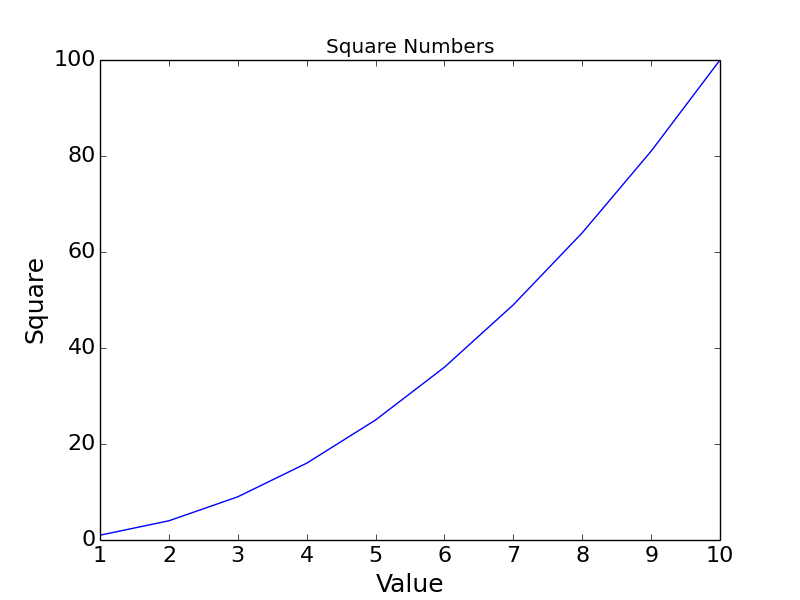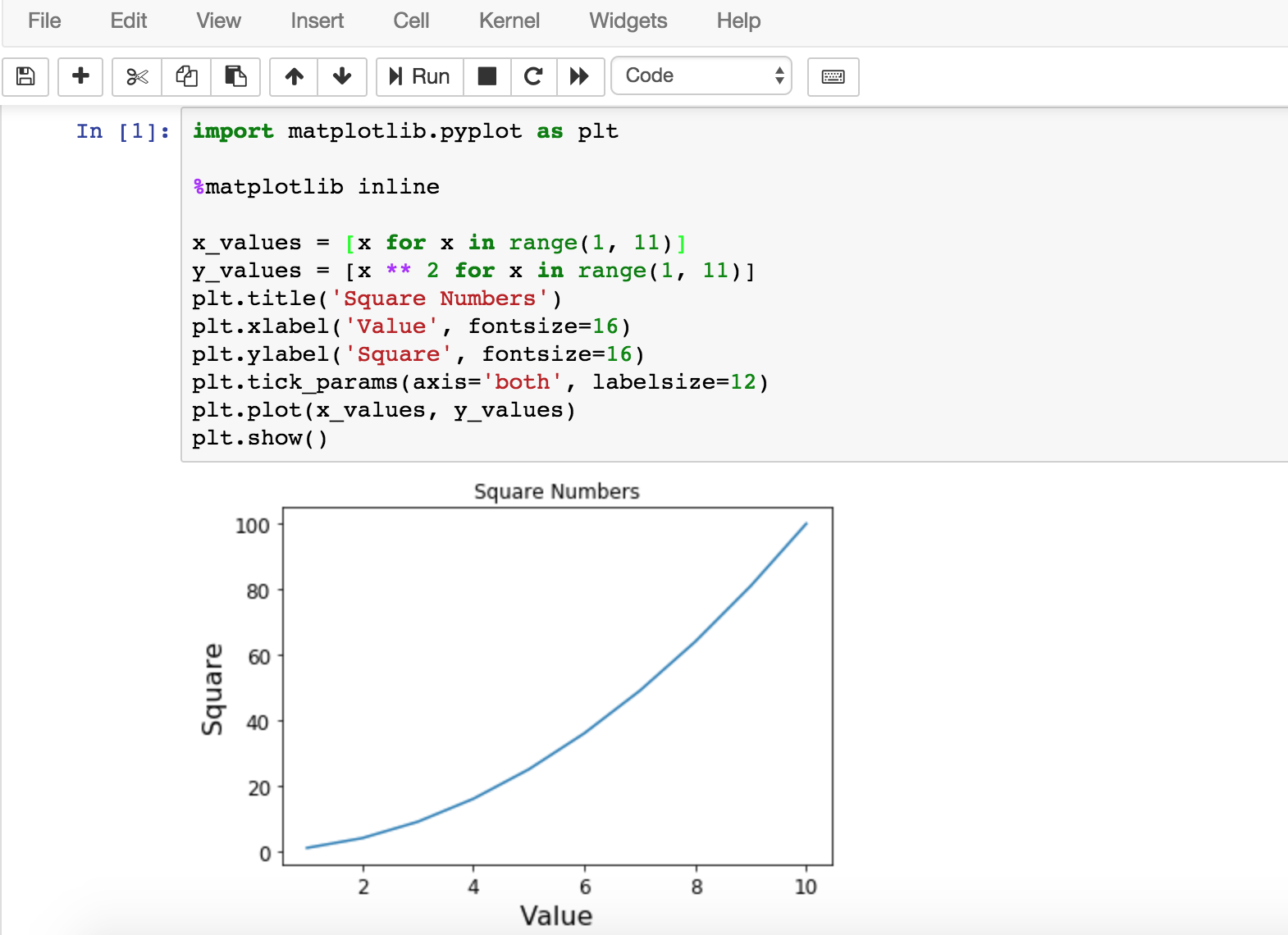### 绘制散点图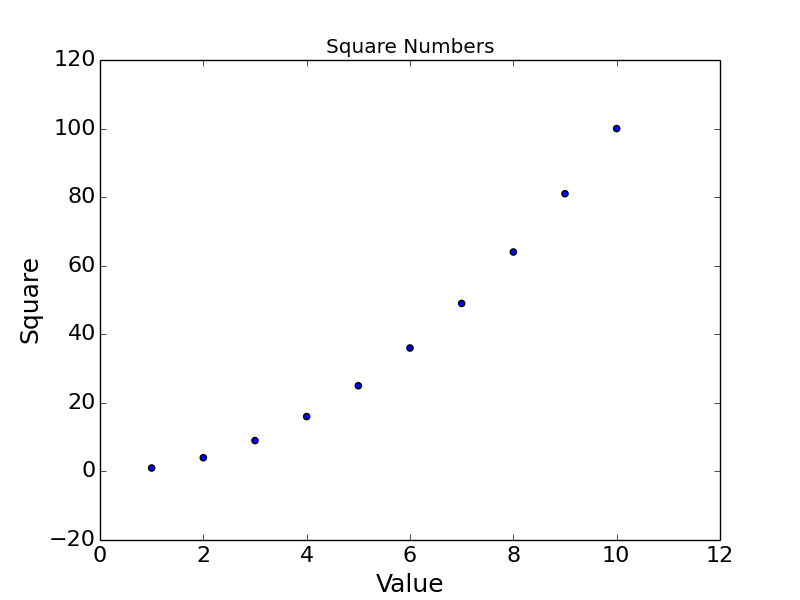plt.plot(x_values, y_values, 'xr')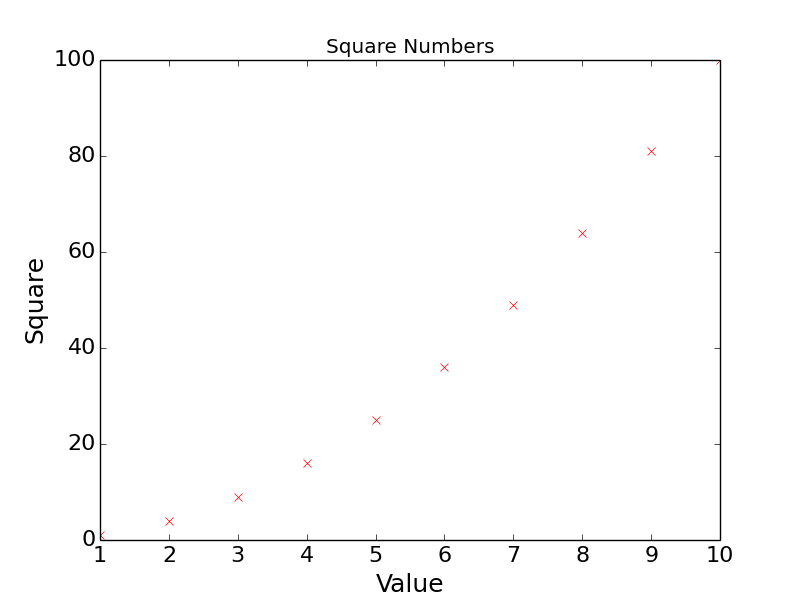plt.axis([0, 12, 0, 120])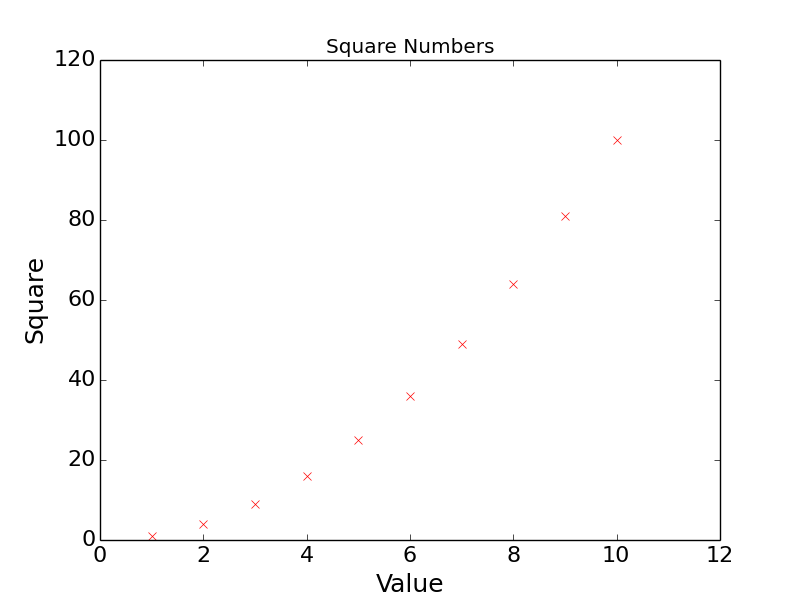### 绘制正弦曲线

# coding: utf-8
import matplotlib.pyplot as plt
import numpy as np

def main():
# 指定采样的范围以及样本的数量
x_values = np.linspace(0, 2 * np.pi, 1000)
# 计算每个样本对应的正弦值
y_values = np.sin(x_values)
# 绘制折线图(线条形状为--, 颜色为蓝色)
plt.plot(x_values, y_values, '--b')
plt.show()

if __name__ == '__main__':
main()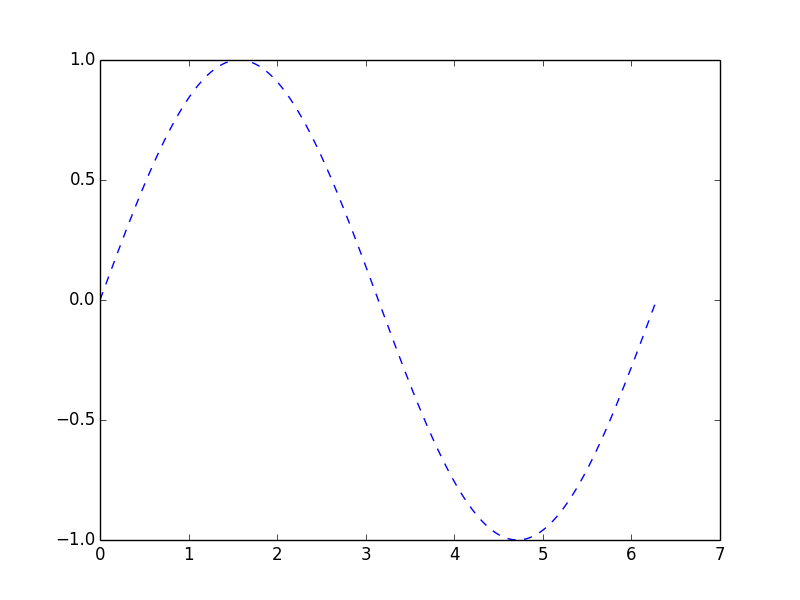# coding: utf-8
import matplotlib.pyplot as plt
import numpy as np

def main():
x_values = np.linspace(0, 2 * np.pi, 1000)
plt.plot(x_values, np.sin(x_values), '--b')
plt.plot(x_values, np.sin(2 * x_values), '--r')
plt.show()

if __name__ == '__main__':
main()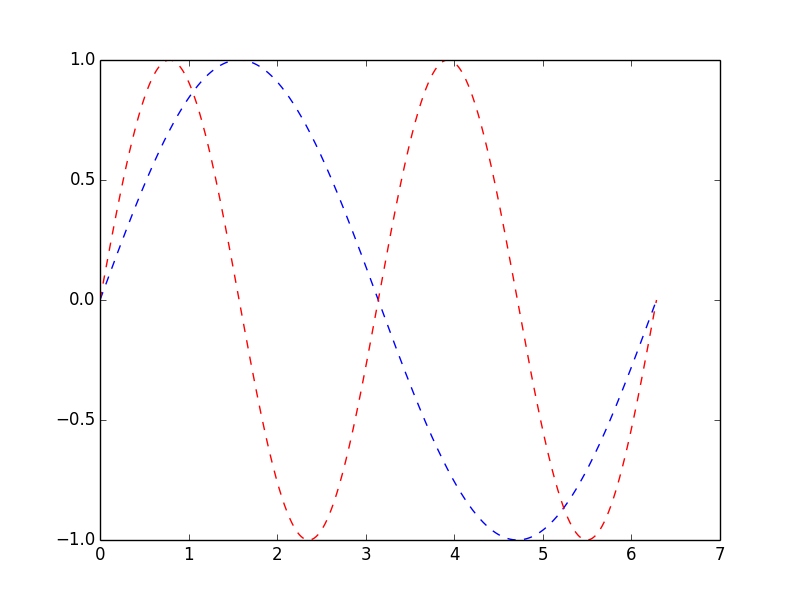# coding: utf-8
import matplotlib.pyplot as plt
import numpy as np

def main():
# 将样本数量减少为50个
x_values = np.linspace(0, 2 * np.pi, 50)
# 设置绘图为2行1列活跃区为1区(第一个图)
plt.subplot(2, 1, 1)
plt.plot(x_values, np.sin(x_values), 'o-b')
# 设置绘图为2行1列活跃区为2区(第二个图)
plt.subplot(2, 1, 2)
plt.plot(x_values, np.sin(2 * x_values), '.-r')
plt.show()

if __name__ == '__main__':
main()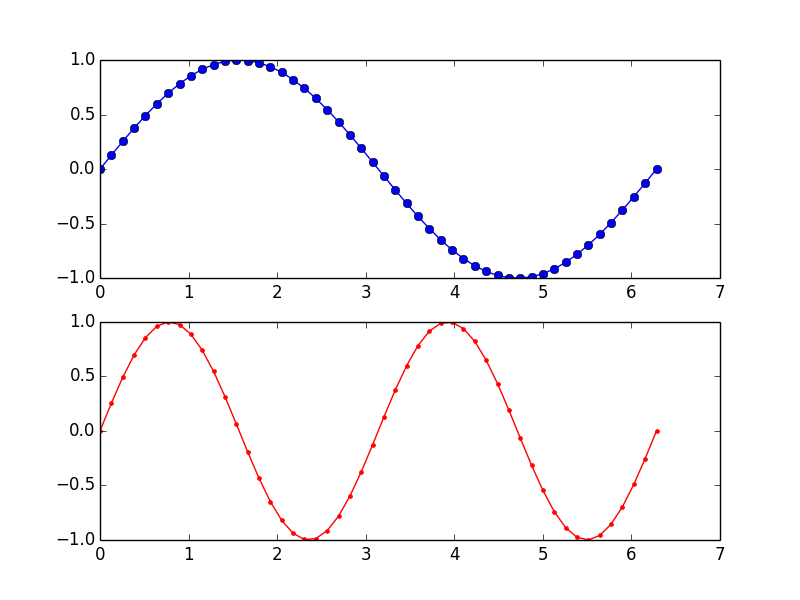### 绘制直方图

# coding: utf-8
import matplotlib.pyplot as plt
import numpy as np

def main():
# 通过random模块的normal函数产生1000个正态分布的样本
data = np.random.normal(10.0, 5.0, 1000)
# 绘制直方图(直方的数量为10个)
plt.hist(data, 10)
plt.show()

if __name__ == '__main__':
main()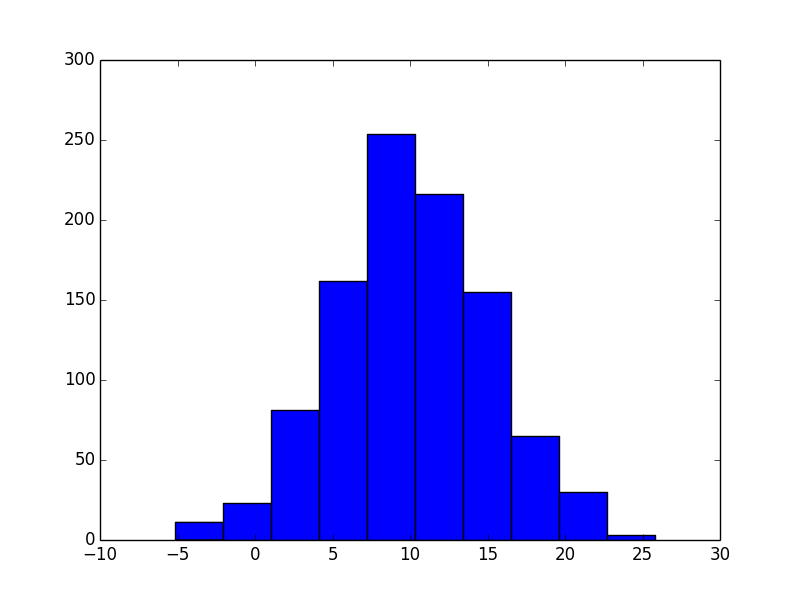### 使用Pygal绘制矢量图

Python中可以使用Pygal来生成SVG，可以通过pip来安装Pygal。

from random import randint
import pygal

def roll_dice(n=1):
total = 0
for _ in range(n):
total += randint(1, 6)

def main():
results = []
# 将两颗色子摇10000次记录点数
for _ in range(10000):
face = roll_dice(2)
results.append(face)
freqs = []
# 统计2~12点各出现了多少次
for value in range(2, 13):
freq = results.count(value)
freqs.append(freq)
# 绘制柱状图
hist = pygal.Bar()
hist.title = 'Result of rolling two dice'
hist.x_labels = [x for x in range(2, 13)]
# 保存矢量图
hist.render_to_file('result.svg')

if __name__ == '__main__':
main()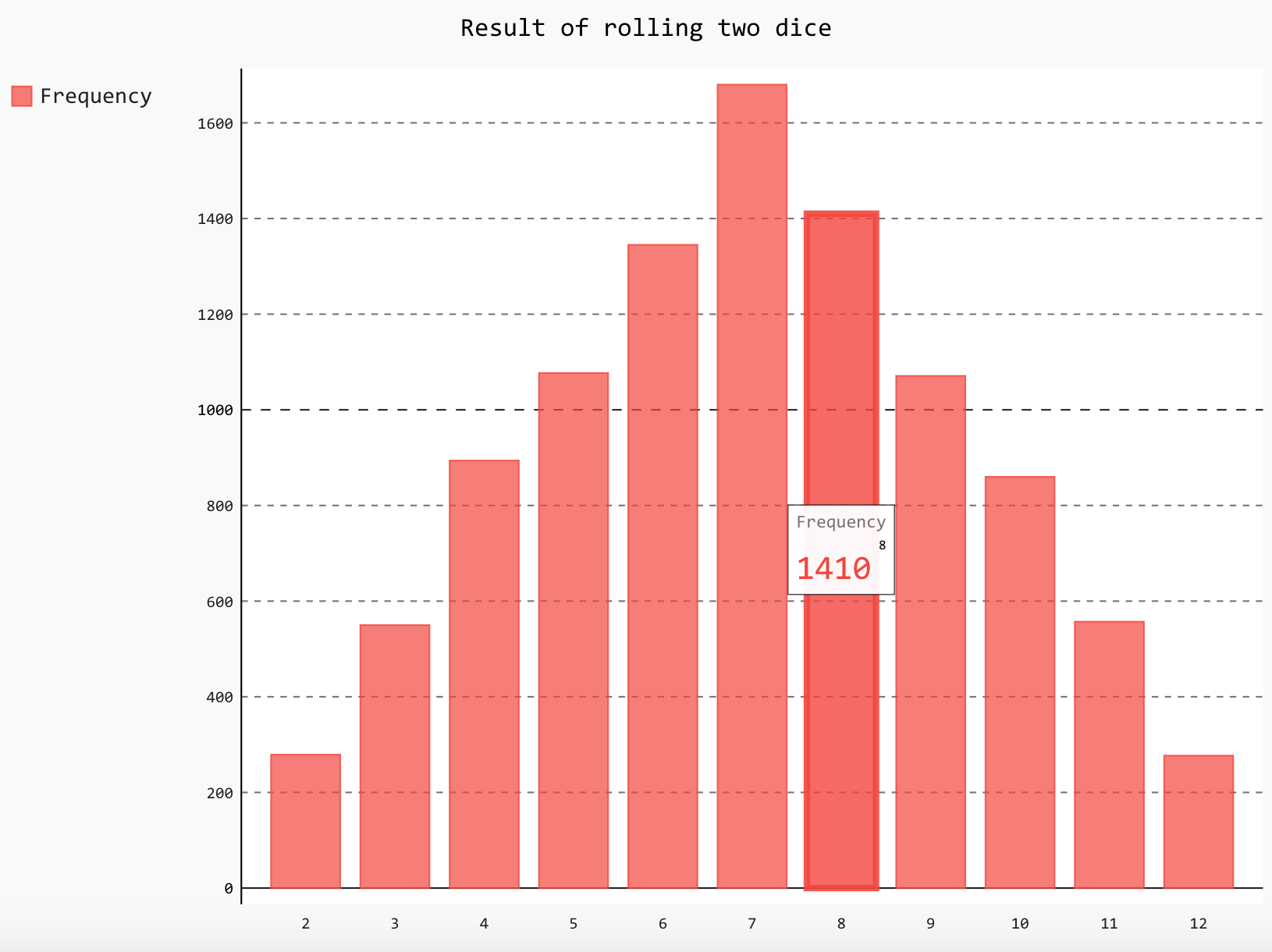### 后记

Matplotlib和NumPy的强大我们在这里也只是窥视了其冰山一角，我们在后续的内容里面还会使用到这两个第三方库，到时候我们再续点为大家介绍其他的功能。

©️2019 CSDN 皮肤主题: 编程工作室 设计师: CSDN官方博客